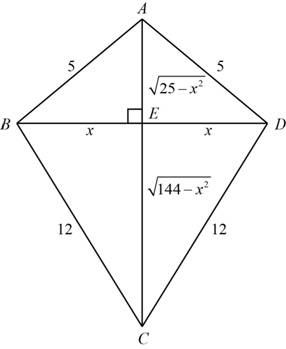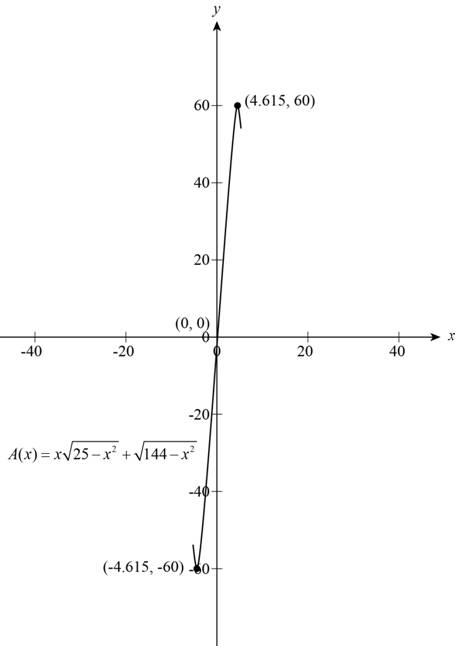# The area function of the kite is A ( x ) = x ( 25 − x 2 + 144 − x 2 ) .### Precalculus: Mathematics for Calcu...

6th Edition
Stewart + 5 others
Publisher: Cengage Learning
ISBN: 9780840068071### Precalculus: Mathematics for Calcu...

6th Edition
Stewart + 5 others
Publisher: Cengage Learning
ISBN: 9780840068071

#### Solutions

Chapter 2, Problem 32P

(a)

To determine

## To show: The area function of the kite is A(x)=x(25−x2+144−x2) .

Expert Solution

### Explanation of Solution

The kite contains four triangles with finite lengths is shown below,Figure (1)

In ΔAEB ,

Use the Pythagoras theorem to find the length AE,

AB2=AE2+BE2[Hyptheses2=base2+perpendicular2](5)2=AE2+x2AE2=25x2

In ΔDEC

Use the Pythagoras theorem to find the length CE,

DC2=EC2+DE2[Hyptheses2=base2+perpendicular2](12)2=EC2+x2AE2=144x2

The formula to calculate the area of triangle is,

Areaof triangle = 12×base×Height

The formula to calculate area of kite,

Areaof kite = Areaof ΔABD+Areaof ΔBCD= 12×2x×25x2+ 12×2x×144x2A(x)=x(25x2+144x2)squareunits

Thus, the area function of the kite is A(x)=x(25x2+144x2) .

(b)

To determine

### To find: The length of the cross pieces of the kite to maximize the area of the kite.

Expert Solution

The length of the two cross pieces are 9.952units and the 11.3757units of each of the cross pieces.

### Explanation of Solution

Given:

The given kite frame to be made from six pieces of wood is shown below,Figure (1)

From the part (a), the area function is A(x)=x(25x2+144x2) .

To find the lengths of the cross pieces of the kite which maximize the area of the kite, it needs to draw the graph of the function A(x)=x(25x2+144x2) .

The function contains the variable x is length of the horizontal cross piece.

The local maximum value of the function is the maximum finite value where the value of the function at the any number is greater than to the original function.

The condition for local minimum is,

f(a)f(x)

The graph of the function is shown below.Figure (2)

From the graph of the function shown as Figure (2) the greatest peak of the graph at the point (4.615,60) .

Then, the maximum area of the kite is 60mi2atx=4.615 .

The length of the side BD in the figure (1) is,

Substitute 4.979 for x in the value of BD=2x .

BD=2x=24.979=9.958

The length of the side AC in the figure (1) is 25x2+144x2 .

Now, substitute 4.979 for x in the length of the side AC.

AC=25x2+144x2=25(4.979)2+144(4.979)2=11.3757

So, the original value of x is 4.979miles .

Thus, the value of one cross piece is 9.952miles and the other crosspiece is 11.3757miles .

### Have a homework question?

Subscribe to bartleby learn! Ask subject matter experts 30 homework questions each month. Plus, you’ll have access to millions of step-by-step textbook answers!